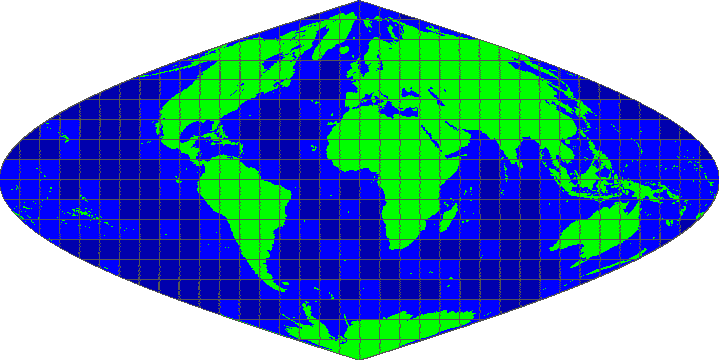# MODIS HDF data extraction in R

The Moderate-resolution imaging spectroradiometer (MODIS) sensors on both Aqua and Terra platforms provide a wealth of (environmental) data. However, manipulating the raw files can be challenging. Although Google Earth Engine provides an easier way to access these data, as most of the MODIS products are hosted, sometimes direct manipulation is still necessary. Here I quickly outline how to extract data from raw MODIS files using R.

## Dependencies

To download extract data from a MODIS HDF file in R you need a few packages. Mainly:

After installing these packages make sure you load them to continue.

require(raster)
require(sp)
require(MODIS)


## Finding your way on the globe

First you have to determine where your data is located within the tiled format MODIS data is distributed in. All tiles are denoted with a horizontal (h) and a vertical index (v). For the Land Products there are roughly ~350 or so tiles with varying degrees of actual land coverage. All MODIS data are distributed in a custom sinusoidal projection, hence the rather weird looking shape of the map.### Tiles

In order to find the right tile we load a coordinate into R. In this case it’s the location of Harvard Yard, or 42.375028, -71.116493 latitude and longitude respectively.

# the location of Harvard Yard
harvard_yard = cbind(42.375028, -71.116493)

# define the projection system to use
# lat-long in this case
latlon = CRS('+proj=longlat +datum=WGS84 +no_defs +ellps=WGS84 +towgs84=0,0,0')

# create a spatial object from the coordinate(s)
coordinates = SpatialPoints(harvard_yard, latlon)

# get the horizontal and vertical tile location for the coordinate(s)
horizontal = extract(tiles,coordinates)$h vertical = extract(tiles,coordinates)$v


Once you have the horizontal and vertical tiles you can download only these tiles instead of the entire archive.

### Extracting data

As previously mentioned, data is stored in HDF files. These files are readily readable by the GDAL library. However, given the structured nature of these files you have to know what you are looking for. These layers in structured HDF files are called scientific data sets (SDS). You need to specify one to read them individually.

# read in all SDS strings (layer descriptors)
# using the MODIS package
sds <- getSds(filename)

# grab layer 4 of a particular hdf file
my_hdf_layer = raster(readGDAL(sds\$SDS4gdal, as.is = TRUE))

# should the HDF file not contain any layers, or a singular one
# you can default to using raster() any additional arguments
my_hdf_file = raster(filename)


Now you have succesfully read in data. However, this data is projected using the sinusoidal projection, not the lat-long. In order to extract data at given locations we have to translate the coordinates of these extraction points to the MODIS sinusoidal projection.

# specify the sinusoidal projection
sinus = CRS("+proj=sinu +lon_0=0 +x_0=0 +y_0=0 +a=6371007.181 +b=6371007.181 +units=m +no_defs")

# transform the coordinates
coordinates_sinus = spTransform(coordinates,sinus)


Finally extract the data at the new sinusoidal coordinates.

# extract data
my_data_point = extract(my_hdf_layer,coordinates_sinus)